# Algebra 1 : How to find the solution to a quadratic equation

## Example Questions

1 2 3 5 Next →

### Example Question #41 : Quadratic Equations

Find the roots of the quadratic equation: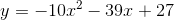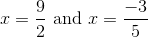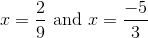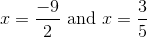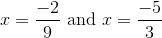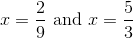Explanation:Reverse Foil Method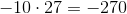Factors of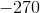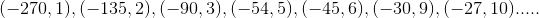Factors that add to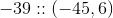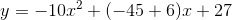Regroup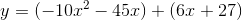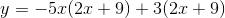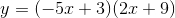Roots occur at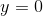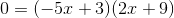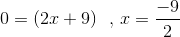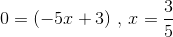### Example Question #41 : How To Find The Solution To A Quadratic Equation

A farmer is building a fence around a field. He knows that the length of the field is 11 meters more than twice its width. If he knows that the area of the field is 30 square meters, what is the perimeter, in meters, of the field?

19

34

30

17

45

34

Explanation:

In order to find the perimeter, start by defining the variables. It is typically easier to define one of the variables in terms of the other; therefore, only one unknown will need to be calculated to find the perimeter. The problem states that the length is eleven more than twice the width; thus, we can define our variables in the following way:The farmer knows that the field's area is thirty square meters. Area is found using the following formula:Substitute in the known value for the area and the defined variables for the length and width.Notice that this equation possesses all the components of a quadratic. Use the information in the equation to construct a quadratic equation that can be factored to obtain an answer. Start by multiplying the first term by the variable on the right side of the equation.In order to make the quadratic equal to zero, subtract 30 from both sides of the equation.Now, factor the quadratic and solve for the variable. We can use the ac method to solve for the variable. Quadratics can be written in the following format:We need to find two numbers whose product equals a multiplied by c and whose sum equals b; therefore, the product of the factors must be -60 and their sum must equal 11. Write out the prime factorization of 60.There is one factor of -60 that when added together sum to equal 11: 15 and -4.Use the factors and split the middle term in the quadratic in order to make factoring by grouping possible.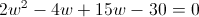Pull the greatest common factor from each pair of terms:from the first and 15 from the second.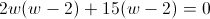Factor out the quantityfrom both terms.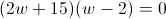Set each factor equal to zero and solve for w.We can cross out the this negative option because the width of a dimension cannot be a negative value. Solve for w in the second factor.The width of the field is 2 meters. Substitute 2 in for the variable and solve for the perimeter.Perimeter is found using the formula:The perimeter of the field is 34 meters.

1 2 3 5 Next →

### All Algebra 1 Resources